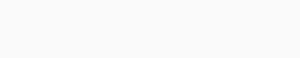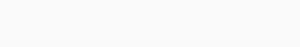Finite Difference is the most popular method to compute numerical derivatives. It is basically the foundation of solving many ordinary and partial differential equations numerically. This method separates the continuous function domain into a finite number of nodes to form a grid. So for a one dimensional problem, like computing derivatives for a univariate problem, it picks a set of points along the x-axis. Similarly, for a two dimensional problem like computing partial derivatives for a bivariate function, it constructs on the plane of the grid.A Grid of Equidistance Nodes chosen for a 2D Function Domain

With all the values of the functions on the grid known, Taylor expansion gives an expression of the derivative that we want to compute in the form of an algebraic equation or a system of algebraic equations and an error term. Finite difference is an approximate numerical solution that transforms a differential problem into an algebraic problem. The mathematical concept is innate and the expression is simple.

There are many ways to construct a finite difference problem using the Taylor expansion. The basic methods are: first-order forward difference, first-order backward difference, first-order central difference, second-order central difference, etc. There are also some explicit methods in which we simply input the numbers to get the results and there are also implicit methods in which we need to solve a system of algebraic equations to get the results. The errors of the different finite differences can be first-order, second-order or higher-order of the grid size.

The first-order derivative of a univariate function is the simplest and the easiest to explain. It can be defined as the slope of the function at the given point. A slope between two points is defined as the quotient, $$\frac{\Delta f}{\Delta x}$$, of the change in the function value between the two points, $$\Delta f$$, divided by the difference of the two points, $$\Delta x$$. The line joining these two pints is called the secant of the function between the two points. When one of the points move towards the other, the secant line moves. When one points overlaps the other or the two points become one, the secant line becomes the tangent line of the function at that point. The slope of that tangent line is defined as the slope of the function at the contact point, hence it is also called the first-order derivative of the function at that point. So, we can say that first order derivative is the quotient of the differences when the step size $$\Delta x$$ is infinitesimally small. When $$\Delta x$$ is very small, the quotient is a very good approximation of the first order derivative $$\frac{df}{dx}$$. We can have different finite differences depending on how we choose the two points.

Forward Difference

Suppose we want to compute the derivative of $$f$$ at $$x_0$$. Consider a linear interpolation between $$(x_0,f(x_0))$$ and $$(x_0+h,f(x_0+h))$$. The slope of the secant line between these two points approximates the derivative by the forward , i.e. two-point difference.

$$\displaystyle f'(x_0)\approx \frac{f(x_0+h),f(x_0)}{h}$$

We can prove the following using the Taylor Expansion.

$$\displaystyle f(x_0+h)=f(x_0)+hf'(x_0)+\frac{h^2}{2!}f”(\xi),x_0\leq\xi\leq x_0+h$$

$$\displaystyle f'(x_0)=\frac{f(x_0+h)-f(x_0)}{h}-\frac{h}{2!}f”(\xi),x_0\leq\xi\leq x_0+h$$

$$\displaystyle f'(x_0)\approx \frac{f(x_0+h)-f(x_0)}{h}$$

The error term is given by:

$$\displaystyle R(x)=||f'(x_0)-\frac{f(x_0+h)-f(x_0)}{h}||=\frac{h}{2!}f”(\xi)=O(h)$$

The big “O” notation implies that the error term is always smaller, i.e. bounded by a constant multiplied by $$h$$. Simply we can say that, the error goes to 0 faster than the constant multiplied by $$h$$. The smaller the $$h$$, the smaller the error term. Then both go to zero at a linear rate. The error term is of first order. Forward difference is useful when solving ordinary differential equations using single-step-predictor-corrector methods.

Backward Difference

Consider a different linear interpolation between $$(x_0-h,f(x_0-h))$$ and $$(x_0,f(x_0))$$. The slope of the secant line between these two points approximates the derivative and is given by the backward, i.e. two-point difference
$$\displaystyle f'(x_0)\approx\frac{f(x_0)-f(x_0-h)}{h}$$

We can prove the following using the Taylor Expansion.

$$\displaystyle f(x_0-h)=f(x_0)-hf'(x_0)+\frac{h^2}{2!}f”(\xi),x_0-h\leq\xi\leq x_0$$

$$\displaystyle f'(x_0)=\frac{f(x_0)-f(x_0-h)}{h}+\frac{h}{2!}f”(\xi),x_0-h\leq\xi\leq x_0$$

The error term is the same as that of the forward difference:

$$\displaystyle R(x)=\Big||f'(x_0)-\frac{f(x_0)-f(x_0-h)}{h}\Big||=\frac{h}{2!}f”(\xi)=O(h)$$

Backward difference is useful when data in the future are not yet available. In certain contro problems, data in the future may depend on the derivatives estimated from the data in the past.

Central Difference

Now consider a  different interpolation between $$(x_0-h,f(x_0-h))$$ and $$(x_0+h,f(x_0+h))$$. The slope of the secant line between these two points approximate the derivative by the central, i.e. three-point difference:
$$\displaystyle f'(x_0)\approx\frac{f(x_0+h)-f(x_0-h)}{2h}$$We can prove the following using the Taylor Expansion.

$$\displaystyle f(x_0+h)=f(x_0)+hf'(x_0)+\frac{h^2}{2!}f”(\xi)+\frac{h^3}{3!}f”'(\xi_1),x_0\leq\xi_1\leq x_0+h$$

$$\displaystyle f(x_0-h)=f(x_0)-hf'(x_0)+\frac{h^2}{2!}f”(\xi)-\frac{h^3}{3!}f”'(\xi_2),x_0-h\leq\xi_2\leq x_0$$

Substracting these two equations, we have

$$\displaystyle f'(x_0)=\frac{f(x_0+h)-f(x_0-h)}{2h}-\frac{h^2}{3!}f”'(\xi_1),x_0\leq\xi_1\leq x_0+h$$

The error term is given by:

$$\displaystyle R(x)=\Big|\Big|f'(x_0)-\frac{f(x_0+h)-f(x_0-h)}{2h}\Big|\Big|=\frac{h^2}{3!}f”'(\xi)=O(h^2)$$

The Central Difference has an error given by $$O(h^2)$$. It means that the error goes to 0 quadratically and faster than it would go linearly. This error term is smaller comparatively from both the forward and backward differences. Central Difference is thus more accurate than forward and backward differences and is therefore the most preferred if data values  are available both in the past and in the future.

for example, if we want to compute the first order derivative of the following function:

$$f(x)=-(x^2-4x+6)$$ at $$x=2$$.

Forward difference gives a negative result because the secant is downward sloping; backward difference gives a positive result because the secant is upward sloping; whereas central difference says that $$\frac{df}{dx}=0$$, which is the correct answer.

If the data points are equally spaced, central difference is the average of the forward and backward differences respectively. Central difference is often used in solving partial differential equations.

Higher Ordered Derivatives

If the first order derivative $$f'(x)$$ of the function $$f(x)$$ is also differentiable, the derivative of $$f'(x)$$ is called the second order derivative of the function $$f(x)$$. Mathematically,
$$f”(x)=(f'(x))’$$
By induction, if the $$(n-1)^{th}$$ order derivative of $$f(x)$$ exists and is differentiable, then the $$n^{th}$$ order derivative is defined as:
$$f^{(n)}(x)=(f^{(n-1)(x)})’$$
If a function, $$f$$ is continuous, we can say that $$f \epsilon C^0$$. If $$f’$$ exists and is continuous, we can say that $$f$$ is a smooth function and $$f\epsilon C^1$$. If $$f$$ can be differentiated indefinitely, we can say that $$f\epsilon C^\infty$$. $$f(x)=e^x$$ is in $$C^\infty$$.
Now we list some of the finite difference formulas for higher order derivatives below.
Second order derivative forward difference:
$$\displaystyle f”(x)=\frac{f(x+2h)-2f(x+h)+f(x)}{h^2}+O(h^2)$$
Second order derivative backward difference:
$$\displaystyle f”(x)=\frac{f(x)-2f(x-h)+f(x-2h)}{h^2}+O(h^2)$$
Second order derivative central difference:
$$\displaystyle f”(x)=\frac{f(x+h)-2f(x)+f(x-h)}{h^2}+O(h^2)$$
Similarly, Third order derivative forward difference:
$$\displaystyle f”'(x)=\frac{f(x+3h)-3f(x+2h)+3f(x+h)-f(x)}{h^3}+O(h^3)$$
Third order derivative backward difference:
$$\displaystyle f”'(x)=\frac{f(x)-3f(x-h)+3f(x-2h)-f(x-3h)}{h^3}+O(h^3)$$
Third order derivative central difference:
$$\displaystyle f”'(x)=\frac{f(x+2h)-2f(x+h)+2f(x-h)-f(x-2h)}{2h^3}+O(h^3)$$
In general, we have the following.

$$n^{th}$$ order derivative forward difference:

##$$n^{th}$$ order derivative backward difference:$$n^{th}$$ order derivative central difference:The matrix term in all of the above equations are the binomial coefficients. each row of the Pascal’s triangle provides the coefficient for each value of $$i$$.

The formulas mentioned above use two point for the second order derivatives and three points for the third order derivatives. There are also some other formulas which use three points for the second order derivatives and four points for the third order derivatives. In general, we can say that by using linear algebra one can construct finite difference approximations which utilize an arbitary number of points to the left and a different number of points to the right of the evaluation point, for any order derivative. This involves solving a linear system such as the Taylor expansion of the sum of those points around the evaluation point best approximates the Taylor expansion of the desired derivative. This is useful for differentiating a function on a grid, where, as one approaches the edge of the grid, one must sample fewer and fewer points on one side.

For example, we will compute the first order derivatives of the function

$$f(x)=-(x^2-4x+6)$$

Analytically, the first order derivative function is

$$\displaystyle \frac{dy}{dx}=-2x+4$$

The following codes computes the first order derivatives of the example above

				
val f: final UnivariateRealFunction f = new AbstractUnivariateRealFunction() {
override public evaluate(double x) {
return -(x * x - 4 * x + 6), // -(x^2 -4x +6)
}
};
double x= 2;

System.out.println("differentiate univariate functions");

UnivariateRealFunction df1_forward = new FiniteDifference(f, 1, FiniteDifference.Type.FORWARD);
double dfdx = df1_forward.evaluate(x); // evaluate at x
System.out.println(String.format("df/dx(x=%f) = %.16f using forward difference", x, dfdx));

UnivariateRealFunction df1_backward = new FiniteDifference(f, 1, FiniteDifference.Type.BACKWARD);
dfdx = df1_backward.evaluate(x); // evaluate at x
System.out.println(String.format("df/dx(x=%f) = %.16f using backward difference", x, dfdx));

UnivariateRealFunction df1_central = new FiniteDifference(f, 1, FiniteDifference.Type.CENTRAL);
dfdx = df1_central.evaluate(x); // evaluate at x
System.out.println(String.format("df/dx(x=%f) = %.16f using central difference", x, dfdx));



The output is:

				
df/dx(x=2.000000) = -0.0000000298023224 using forward diffference
df/dx(x=2.000000) = 0.0000000298023224 using backward diffference
df/dx(x=2.000000) = 0.0000000000000000 using central diffference



In general, for finite difference, the more points that we use in computing a derivative, the more accurate it is. Also, the higher an order of a derivative, the less accurate it is. For example, the second order derivative is less accurate than approximating the first order derivative.